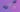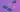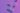# Conditional Statements in Python

This blog explains conditional / decision making/branching statements in python, along with syntax and examples.

In day to day life, we need to make many decisions. When we want to find out the solution of real-life problems through programming then also we need to make many decisions. For this, we have conditional statements in python. Conditional statements can check one condition at a time, and executes the block of code accordingly. We will discuss everything in detail in this blog.

There are some control flow statements in Python. Control flow refers to the order in which the program should be executed. Generally, the control flow of a program runs from top to bottom.

However, the control flow statements break the general top-to-bottom order of execution by including decision-making, looping, and more. This enables the program to first execute a specific block of code based on the conditions used.

In this blog, we will learn if else control flow statements, the list of topics is as given below:

• Python Conditional Statements
• if else in Python
• if elif else Python
• Nested if in Python
• Shorthand If and If else in Python

Python Conditional Statements

Decision-making in programming, much similar to decision-making in real life, is quite important as it helps us decide what the program should do next. Decision-making helps in deciding the flow of execution of the program. Decision-making is implemented using if else in Python. For conditional logic in python “if else” structure is used.

## If in Python

Starting from the if statement, it is the most basic decision-making statement. It simply decides whether a particular code block will be executed or not on the basis of the condition provided in the if statement. If the condition provided in the if the statement is true, then the code block is executed, and if it’s false then the code block is not executed.

The diagram given below shows the flow of execution of if statement in python.Syntax of if statement in Python:

if expression:

statement(s)

As given in the syntax above, the Python program first evaluates the condition or expression. If the condition is met or if the condition is true, then only the statement(s) in the body of the if the statement is (are) executed. If the condition is false then the block will be skipped and the control will directly go to the next statement after the block.

Note The body of the if statement in Python starts after an indentation, unlike other languages that use brackets to write the body of if statements. In python, indentation is specified by using 4 white spaces.

Example of if statement in Python:

a = 0

if (a <5):

print (“0 is less than 5”)

print(“This is the statement after if statement”)

Output:

0 is less than 5

This is the statement after if statement

## If else in Python

If the statement tells the program what to do when if the condition is true. In case the condition is false, the program just goes on to execute what comes after if statements. In situations where we want the program to execute some statement if the condition is true and some other states only if the condition is false, then we use if-else statement in Python.

The diagram given below shows the flow of execution of if-else statement in python.As shown in diag. above, the Python program first evaluates the test expression. The test expression is the condition in the if statement. If the condition is met or if the condition is true, then only the statement(s) in the body of the if the statement is(are) executed. If the condition is not true, then the statement in the body of the else statement is executed. The body of if else statements start with indentation.

Syntax of if else in Python:

if test expression:

Body of if

else:

Body of else

Example of if…else statement.

i = 20

if (i < 15):

print (“i is smaller than 15”)

else:

print (“i is greater than 15”)

print (“statement after if statement”)

Output:

i is greater than 15

statement after if statement

## IfElif Else in Python

Here, the elif stands for else if in Python. We have seen that we can check one or two conditions using if and if else statements in python. But what if I want to check multiple conditions? We can do it in python using conditional statement. Typically speaking using if elif else statement.

If the first if condition is true, then same as in the previous if and if else statements, the program will execute the body of the if statement. But if the first condition is false then, the program will go to the elif block (else if in Python) which basically checks for another if statement. Again, if the condition is true, the program will execute the body of the elif statement, and if the condition is false, then the program control will go to the next else statement and the body of else block will get executed.

Diagram given below shows the flow of execution of if elif else statement in python.When there are more if conditions than two conditions in if elif else statements, then it is referred to as if elif else ladder, as it resembles the structure of a ladder in terms of if statements. When any one of the condition is true, then remaining ladder is just skipped and the body of that particular if block is executed. If all the if conditions turn are false, then the body of the last else block will get executed.

Syntax of the if elif else in Python

if test expression:    Body of ifelif test expression:    Body of elif..else:    Body of else

In if elif else ladder of python we can put any number of elif statements as per requirement of our program. We can add it before the last else statements, making it an if elif else ladder.

Example of the if elif else statement:

a = 50if (a == 20):   print (“value of variable a is 20”)elif (a == 30):    print (“value of variable a is 30”)elif (a == 40):    print (“value of variable a is 40”)else:    print (“value is greater than 40”)    Output:value of variable a is greater than 40

In the above example, the program first checks the very first if statement. Since it turns out to be false, the body of the if statement is not executed, and the program goes to the next elif statement. When the condition of first elif is checked, that condition also turns out to be false and again the body of the elif block is skipped, and program goes to the next elif statement. Same thing happens here. As all conditions were false, the program finally reaches the last else statement and executes the body of else. So, we will get the output as ‘value of variable a is greater than 40’.

## Nested If in Python

As the name suggests, nested if statement means one if statement inside another if statement. Means in nested if statement, we have one if as the body of another if statement. Nested if statements are used when we want to check secondary conditions only if the first condition are true.

Syntax of nested if in Python:

if test expression 1:

# Executes when condition 1 is true

body of if statement

if test expression 2:

# Executes when condition 2 is true

Body of nested if

else:

body of nested if

else:

body of if else statement

Example of if in Python:

a = 20

if (a == 20):

# First if statement

if (a < 25):

print (“a is smaller than 25”)

else:

print (“a is greater than 25”)

else:

print (“a is not equal to 20”)

Output:

a is smaller than 25

Here in this example of nested if in Python. In the beginning of program value of a is initialized as 20(a=20), the program enters in the first if statement. Then it checks the nested if statements and executes it as true and prints that a is smaller than 25 on the screen.

Now, in the nested if else the else statements won’t be executed, and the program will go to the statement after the end of the if block. That is the working of nested if condition in Python works.

## Shorthand If and If Else in Python

Shorthand if and if else is nothing but a way to write the if statements in one line when we have only one statement to execute in the if block and the else block.

An example for shorthand if in Python:

a = 4

b = 2

if a>b: print(” a is greater than b”)

Output:

a is greater than b

An example for shorthand of state else condition:

x = 4

y = 2

print(“x is greater than y”) if x>y else print (“y is greater than x”)

Output:

x is greater than y

With this, we have come to the end of this blog.  In this blog we have discussed Python conditional statements, if condition in Python, if elif Else Python, nested if statement in Python, shorthand if condition in Python and Python shorthand if else statement.

If you want to know about data types and variables in python, please go through our blog:

Data Types and Variables in Python

Author

Name: Nikita Jagtap

Other Details are as follows:

Designation: Python Trainer